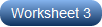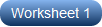# Multiplying Fractions with Cross Cancelling Worksheets

Multiplying fractions with cross cancelling worksheets should be your pick, if the traditional way of multiplying large numbers muddles your grade 6 and grade 7 learners. Break down both the numerator and denominator of each fraction into their prime factors and cross out common factors that appear in both the numerator and denominator, multiply what's left over. Cross cancellation eliminates the need to reduce your answers. Add a twist to the practice of your students with the inclusion of the mixed number in these printable worksheets. Our answer keys take the hassle out of the tedious evaluation process. Wait no longer, print the free multiplying fractions with cross cancelling worksheet and get started.

Multiplying Two Fractions

When's the last time you struggled to halve a recipe that calls for 3/4 of a cup of flour? Direct grade 6 and grade 7 students to cross-cancel, multiply the leftovers in the numerators and denominators and convert to mixed numbers if improper.Multiplying Fractions and Mixed Numbers | Two Terms

Go beyond multiplying two fractions and use the strategy of cross cancelling to multiply a fraction by a mixed number or two mixed numbers. Precede the process with converting the mixed numbers to improper fractions and then cross cancel.Multiplying Three Fractions

Count on these finding the product of three fractions worksheet pdfs for a repeated exposure. Simplify the fractions by cross cancelling and then multiply what is left uncancelled to find the product and convert to mixed fraction, if required.Multiplying Fractions and Mixed Numbers | Three Terms

Take the practice of 6th grade and 7th grade learners to new heights as they convert the mixed numbers to improper fractions and then find all the factors the numerators share with the denominators, cancel them and multiply the uncancelled parts.